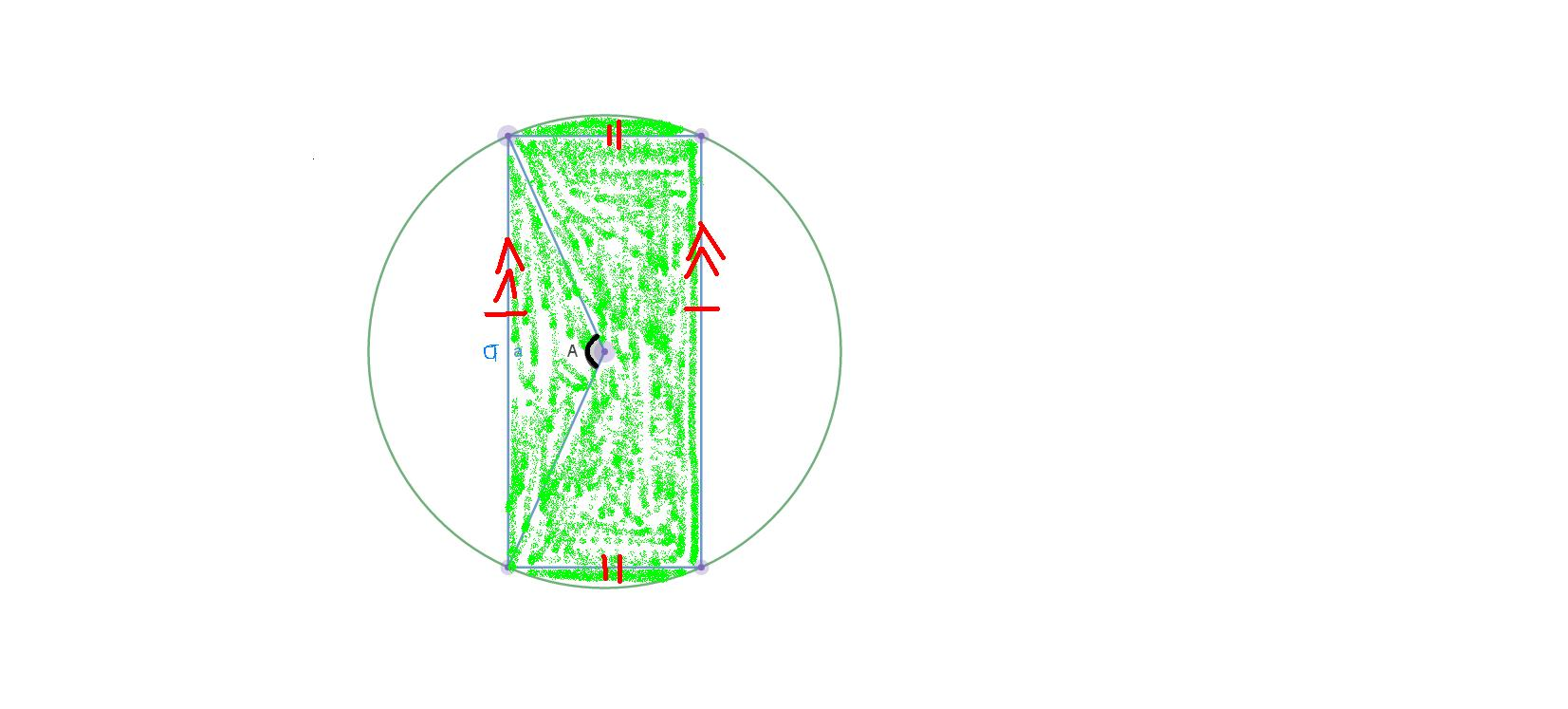# Geometry with approximationsGiven: $\text{Area of the shaded region (green) } = \dfrac{1}{4} \times \text{Area of the whole circle} \text{ (Diagram not to scale)}$

$\text{The vertical lines(each length a) are drawn parallel and equidistant from the axis of symmetry of the figure.}$

$\text{The angle A is between two radii of the circle joining with the left line.}$

$\text{Let R = radius of the circle}$

$\dfrac{1}{2} \times (R)^2 \times (A) - \dfrac{1}{2} \times (R)^2 \times \sin(A) = \dfrac{1}{2} \cdot \dfrac{3}{4} \times \pi (R)^2$ $\text{[Region of one unshaded part]}$

$(A) - \sin(A) = \dfrac{3\pi}{4}$

$\sin(A) = A - \dfrac{3\pi}{4}$

$a = 2 \times \sin{ \left( \dfrac{A}{2} \right) } \times R$

Perimeter of the unshaded region $= 2 \times ( a + (R) (A) )$

$\text{Using (3rd order) Taylor Series approximation:}$

$A - \dfrac{A^3}{3!} \approx A -\dfrac{3\pi}{4}$

$A^3 \approx \dfrac{9\pi}{2}$

$A \approx 2.417988 \text{ rad}$

$\text{Perimeter of unshaded region} \approx 2 \times \left[ \left( 2 \times \sin{ \left( \dfrac{2.417988}{2} \right) } \times R \right) + ( 2.417988)(R) \right]$

$\text{Perimeter of unshaded region} \approx 8.57702 R$

$\text{Note : The answer is inaccurate because the approximation only works well near }$

$x = 0$. $\text{ Nevertheless, Taylor Series is useful to approximate difficult calculations.}$Note by Lin Shun Hao
1 year, 1 month ago

This discussion board is a place to discuss our Daily Challenges and the math and science related to those challenges. Explanations are more than just a solution — they should explain the steps and thinking strategies that you used to obtain the solution. Comments should further the discussion of math and science.

When posting on Brilliant:

• Use the emojis to react to an explanation, whether you're congratulating a job well done , or just really confused .
• Ask specific questions about the challenge or the steps in somebody's explanation. Well-posed questions can add a lot to the discussion, but posting "I don't understand!" doesn't help anyone.
• Try to contribute something new to the discussion, whether it is an extension, generalization or other idea related to the challenge.
• Stay on topic — we're all here to learn more about math and science, not to hear about your favorite get-rich-quick scheme or current world events.

MarkdownAppears as
*italics* or _italics_ italics
**bold** or __bold__ bold
- bulleted- list
• bulleted
• list
1. numbered2. list
1. numbered
2. list
Note: you must add a full line of space before and after lists for them to show up correctly
paragraph 1paragraph 2

paragraph 1

paragraph 2

[example link](https://brilliant.org)example link
> This is a quote
This is a quote
    # I indented these lines
# 4 spaces, and now they show
# up as a code block.

print "hello world"
# I indented these lines
# 4 spaces, and now they show
# up as a code block.

print "hello world"
MathAppears as
Remember to wrap math in $$ ... $$ or $ ... $ to ensure proper formatting.
2 \times 3 $2 \times 3$
2^{34} $2^{34}$
a_{i-1} $a_{i-1}$
\frac{2}{3} $\frac{2}{3}$
\sqrt{2} $\sqrt{2}$
\sum_{i=1}^3 $\sum_{i=1}^3$
\sin \theta $\sin \theta$
\boxed{123} $\boxed{123}$

## Comments

There are no comments in this discussion.

×

Problem Loading...

Note Loading...

Set Loading...